## Mastering Important Concept Test by GMAT in Triangle - II

We invite all community contributors to share resources that are deemed to help students beat the GMAT. We've created individual threads to match the main topics, so please select the best fit for your content carefully.

### GMAT/MBA Expert

GMAT Instructor
Posts: 645
Joined: 26 Jul 2010
Location: US
Thanked: 527 times
Followed by:227 members

### Mastering Important Concept Test by GMAT in Triangle - II

by e-GMAT » Mon Dec 12, 2016 12:02 am
Added the PDF of the article at the end of the post!Mastering Important Concepts Tested by GMAT in Triangles - II

In this article, we will continue looking at the different properties of a triangle. Also, links of two practice questions are given in the next post. These questions are specifically designed to help you apply the learning from this article.

In the previous article on triangles, we discussed the following aspects. In case you haven't gone through the previous article, click here.

• 1. Identifying whether a set of given numbers could be the lengths of the three sides of a triangle,

2. Range of possible values of the length of one side of a triangle given the lengths of the other two sides, and

3. Various properties of the angles of a triangle.

With the above knowledge in mind, let us now look at the different types of triangles and understand how do their properties differ from one another.

Then we will then apply the learning on two GMAT like questions.

1. Properties of Different types of triangles

The table given below lists down the various types of triangles and their properties that are tested on the GMAT. The triangles are sequenced in such a way that the transitions/subtle differences in the corresponding properties are clearly visible.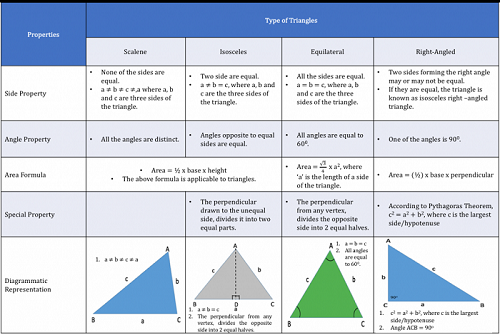1.1 Side property:

• None of the sides of a scalene triangle is equal.

Any two sides of an isosceles triangle are equal.

All the sides of an equilateral triangle are equal.

Note:

A right-angled triangle can be a scalene triangle or an isosceles triangle. Its depends on whether the lengths of the perpendicular and the base are equal.

Can you tell why can't a right-angled triangle be an equilateral triangle?

1.2 Angle Property:

• o None of the angles of a scalene triangle are equal.

o The two angles opposite the two equal sides of an isosceles triangle are equal.

o All the angles of an equilateral triangle are equal.

Can you notice the relation between the sides and the angles?
• "¢ In a scalene triangle where none of the sides are equal, no two angles are equal
"¢ In an isosceles triangle where two sides are equal, the angles opposite to the equal sides are equal.
"¢ In an equilateral triangle where all sides are equal, all angles are equal to 60 degrees.

Note:

A right-angled triangle has one of its angles as 90 degrees. Since it can be a scalene triangle or an isosceles triangle, the value of the other two angles will depend on what kind of triangle it is.
(More will be discussed on this in the third section of the article)

1.3 Area Property

The area of any triangle can be found using the formula -
• Area = Â½ x base x height
(as shown in the diagram)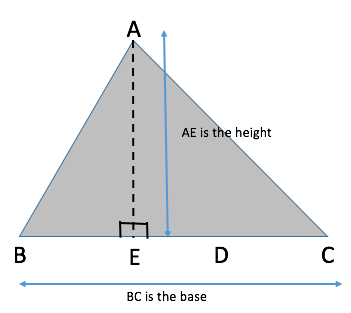For an Equilateral triangle we sometimes use the formula given below to calculate the area quickly: (please note that in an Equilateral triangle all sides and angles are equal)
• Area = (âˆš3/4) x a^2
Where a is the length of each side of the triangle.

Note:

The area of an equilateral triangle or any special triangle is derived from the basic formula - Area = Â½ x base x height.

Thus, if we remember this formula, it is more than enough to solve any problem related to finding the area of a triangle.

1.4 Special Property of Triangles

1.4.1 Isosceles Triangle

The line joining one vertex of a triangle to the midpoint of the opposite side is called a median of the triangle.

In the case of an isosceles triangle, the median and the perpendicular is the same, when drawn from the vertex which joins the two equal sides.

Hence, from the given diagram, we can say that line AD acts as a median, dividing the base BC into two equal halves, and that AD is also perpendicular to BC.

1.4.2 Right Angled Triangle

The Right Angled Triangle has three sides known as the perpendicular, base and hypotenuse. The hypotenuse is the largest side and the angle opposite to it is the largest angle and measures 90 degrees.

We can apply Pythagoras Theorem, to define the relation between perpendicular, base and hypotenuse.

From the given diagram, we can write:

(Hypotenuse)^2 = (perpendicular)^2 + (base)^2

AC^2 = AB^2 + BC^2

The summary of these concepts is given in a tabular form at the top of the article. Let us now, with the help of two GMAT like questions understand how to apply the above-mentioned properties.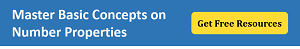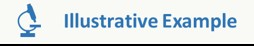In triangle ABC, is AC^2 = AB^2 + BC^2?
• I. âˆ BAC + âˆ ACB = âˆ ABC
II. AB = BC
Explanation:

Steps 1 & 2: Understand Question and Draw InferencesTo find: Is AC^2 = AB^2 + BC^2?

Using Pythagoras theorem, we know that the above is true if âˆ ABC=90
• "¢ Because we can apply the property that we have learned from the table given above that AC^2 = AB^2 + BC^2 only when ABC is a right-angled triangle.

Step 3: Analyze Statement 1 independently

Statement 1 tells us that âˆ BAC + âˆ ACB = âˆ ABC

"¢ Using Angle Sum Property in triangle ABC, we can write:
âˆ BAC+âˆ ACB+âˆ ABC=180

"¢ Substituting the value of âˆ BAC + âˆ ACB from Statement 1, we have Â
âˆ ABC+âˆ ABC=180

2(âˆ ABC) = 180

Therefore, âˆ ABC= 90

"¢ Since we could find a unique answer to the question, Statement 1 alone is sufficient.

Step 4: Analyze Statement 2 independently

Statement 2 tells us that AB = BC

Using the table given above, we can see that this is a property of an isosceles triangle.
"¢ Thus, triangle ABC is an isosceles triangle.

However, we cannot conclude that ABC is a right-angled triangle because not every isosceles triangle is right-angled.

Hence, this statement is clearly not sufficient to solve the question. Therefore, Statement 2 is not sufficient.
Â
Step 5: Analyze Both Statements Together (if needed)

Since we've already arrived at a unique answer in Step 3, this step is not required.The area of an equilateral triangle is âˆš3 times the area of an isosceles triangle. If the three sides of the isosceles triangle are 5,5 and 6. Find the length of each side of the equilateral triangle.
• A. 4
B. 6
C. 4âˆš3
D. 8
E. 6âˆš 3

Explanation:

From the triangle property table, we can use two properties to solve this question.

Isosceles Special Property: The perpendicular drawn to the unequal side, divides the unequal side into 2 equal halves.

Let's name the isosceles triangle as ABC, where AB = AC = 5 units and BC = 6 units.

The perpendicular from A on BC, will divide BC into 2 equal halves. So BD = DC = 3 units

Using Pythagoras Theorem, we can write AB^2 = AD^2 + BD^2

Area of triangle ABC = Â½ x AD x BC = Â½ x 4 x 6 = 12 units.

Area of an equilateral triangle = (âˆš3/4) x side^2

Since it is given that area of equilateral triangle = âˆš3 x area of isosceles triangle

Therefore,

(âˆš3/4) x side^2 = âˆš3 x 12

Side = 4âˆš3

Hence, the correct answer is Option C.

We hope these questions helped you in understanding the importance of the properties of triangles and their application in GMAT context.

Try to remember these properties in a methodical manner as given in the table and we are sure that you will be able to apply them successfully in all questions related to triangles.

Link to the other articles in this series :

Thanks,
Saquib
Quant Expert
e-GMAT

Link to the other articles in this series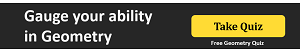Attachments
Triangles_Part2_BTG.pdf